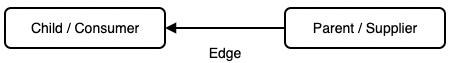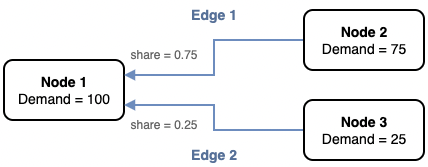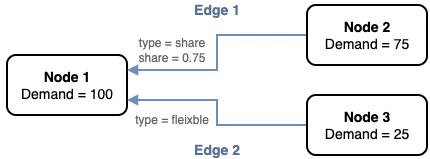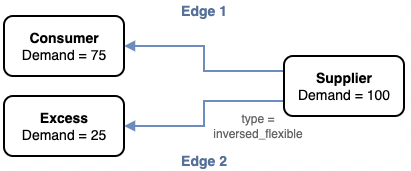# Graph components

Energy flows in the ETM are modelled using a directed graph structure.

Graphs are collections of Nodes, which we typically use to represent the transformation of energy from one form to another, or technologies. Nodes are connected to other nodes by Edges, which we typically use to represent the flow of energy between nodes.A simple graph in which energy flows from right to left, from the supplier (or "parent") to the consumer ("child").

## Carriers​

A "carrier" can be thought of as a type of energy. For example, coal, electricity, wind, and gas are all different energy carriers. Each carrier has a collection of attributes which describe whether it is a sustainable source of energy, the CO2 emissions per MJ, and more.

## Nodes​

Nodes are extremely versatile. They can represent a single technology type or industrial process or can be used more abstractly to combine energy flows from multiple sources to easily query the graph.

For example, the `transport_car_using_electricity` node represents electric cars used for transport. This sends energy into a `transport_useful_demand_cars` node which does not have a real-world counterpart. Instead, it is used to represent the total demand for car-based transport in the graph. Car demand then feeds into `transport_useful_demand_passenger_kms` which represents the total passenger kilometres travelled by all transport methods, including bicycles, busses, cars, and more.

## Edges​

Edges allow us to move energy from one node to another. The ETM uses a directed graph, which means that the edges have a direction; energy flows in one direction through the edge. In the example at the top of the page, energy flows from Node 1 to Node 2 through the edge, but not from Node 2 to Node 1.

Edges are also associated with a carrier, for example electricity.

An edge can only have one carrier, but a node can have multiple edges with different carriers. For example, a node might have an edge for electricity, and another edge for gas.

### Edge shares​

Edges have a `share`, which describes what proportion of the energy of a node is received through the edge. For example, a node might have two electricity edges.75% of the energy in Node 1 is supplied by Node 2, and 25% by Node 3

### Edge types​

In the ETSource document for an edge, you will assign a `type` attribute.

#### Share edges​

Share edges allow you to directly define the share of an edge: the proportion of the supply of a node which is sent to the consumer node. In the ETSource document, you will set this with the `parent_share` attribute; in ETEngine it is simply called `share` for historical reasons.

``- type = share- child_share = 0.75``

Share edges are the most common type used in the ETM.

#### Flexible edge​

Flexible edges are similar to share edges, except that you don't need to explicitly define the share. Instead, because the shares on a node should always sum to 1.0, a flexible edge share will be set to the remaining share after all other edges have been assigned.

``- type = flexible``As in the previous example, Node 1 receives 75% of its energy from Node 2. As the Node 3 edge is flexible, it's share is set dynamically to 1.0 - 0.75 = 0.25.

#### Constant edges​

Constant edges are used extremely infrequently and allow the (ab)use of the `share` document attribute to instead set a specific amount of energy carried through the edge.

``- type = constant- child_share = 100.0``

The edge will carry exactly 100 MJ of energy. Constant edges are often used without specifying the `share`, in which case the edge will carry the full amount of energy from the consumer node.

#### Dependent edges​

Dependent edges are a legacy feature, and behave the same as a constant edge without a `share` (i.e. they carry the full amount of energy from the consumer node).

``- type = dependent``

#### Inversed flexible edges​

Whereas most edges in the graph are calculated from left to right (the "child" node to the "parent"), inversed flexible edges do the opposite. Inversed edges are used to carry excess energy away from a node to a different consumer.75% of the supplier's energy is given by the Consumer, with the remaining 25% sent to the Excess node.

### Reversed edges​

While most edges in the graph are calculated from left to right (the "child" node to the "parent"), reversed edges do the opposite. Reversed edges are used to move energy from the right to the left.

``- type = share- reversed = true``

When a share is assigned to this edge, it will refer to the share of the producer node, rather than the consumer node.

## Slots​

So far, when describing shares we have only discussed examples that use one carrier. However, a node can have multiple edges with different carriers. For example, a node might have an edge for electricity, and another edge for gas.

Edges shares describe the share of the carrier, rather than the share of all the demand on a node.

The above example may be confusing: how can both edges have a share of 1.0? The answer is that each edge takes 100% of the energy of its carrier. The electricity edge carries 100% of the electricity, and the gas edge carries 100% of the gas.

This example shows the situation more clearly. The electricity edge still has a share of 1.0 and carries 100% of the electricity, but the gas edges have shares of 0.75 and 0.25; one carries 75% of the gas, and the other 25%.

However, this example presents another problem: even though we know the proportion of each carrier that passes through each edge, we don't know the proportion of gas to electricity. This is where slots come in.

In ETEngine, nodes have input and output slots which correspond with the input and output edges and carriers. A node with electricity and gas input edges will have an electricity input slot and a gas input slot. A node with an outgoing heat edge will have a heat output slot.

Like edges, each slot also has a `share` which describes the proportion of the node's total demand which is carried by the slot.

In ETSource, the shares of the slots are set with `input` and `output` attributes:

``- input.electricity = 0.6- input.gas = 0.4- output.heat = 1.0``

In ETEngine, these shares can be queried with `conversion` attributes on the node:

``V(Consumer, electricity_input_conversion) # => 0.6V(Consumer, gas_input_conversion)         # => 0.4V(Consumer, heat_output_conversion)       # => 1.0``